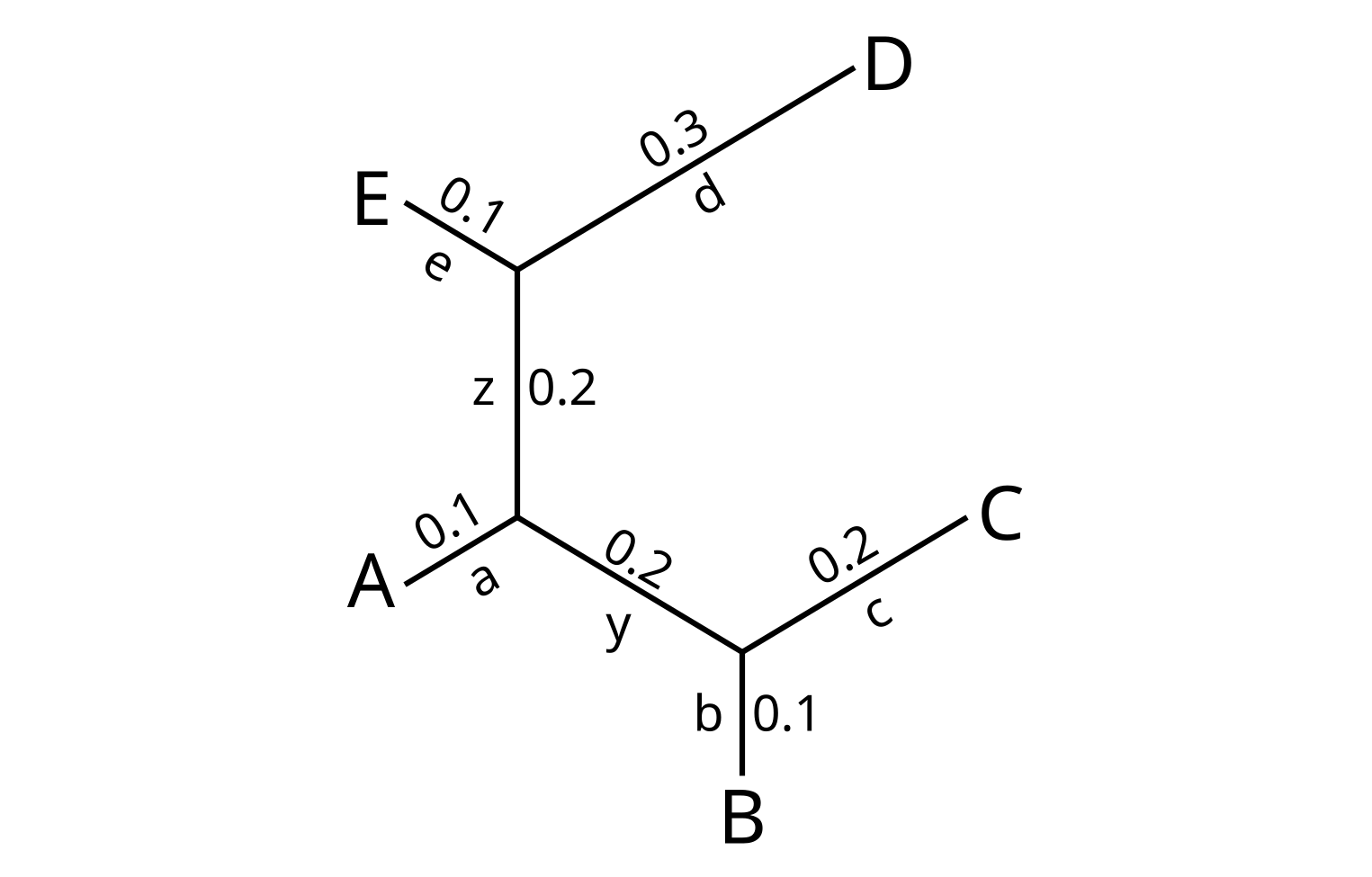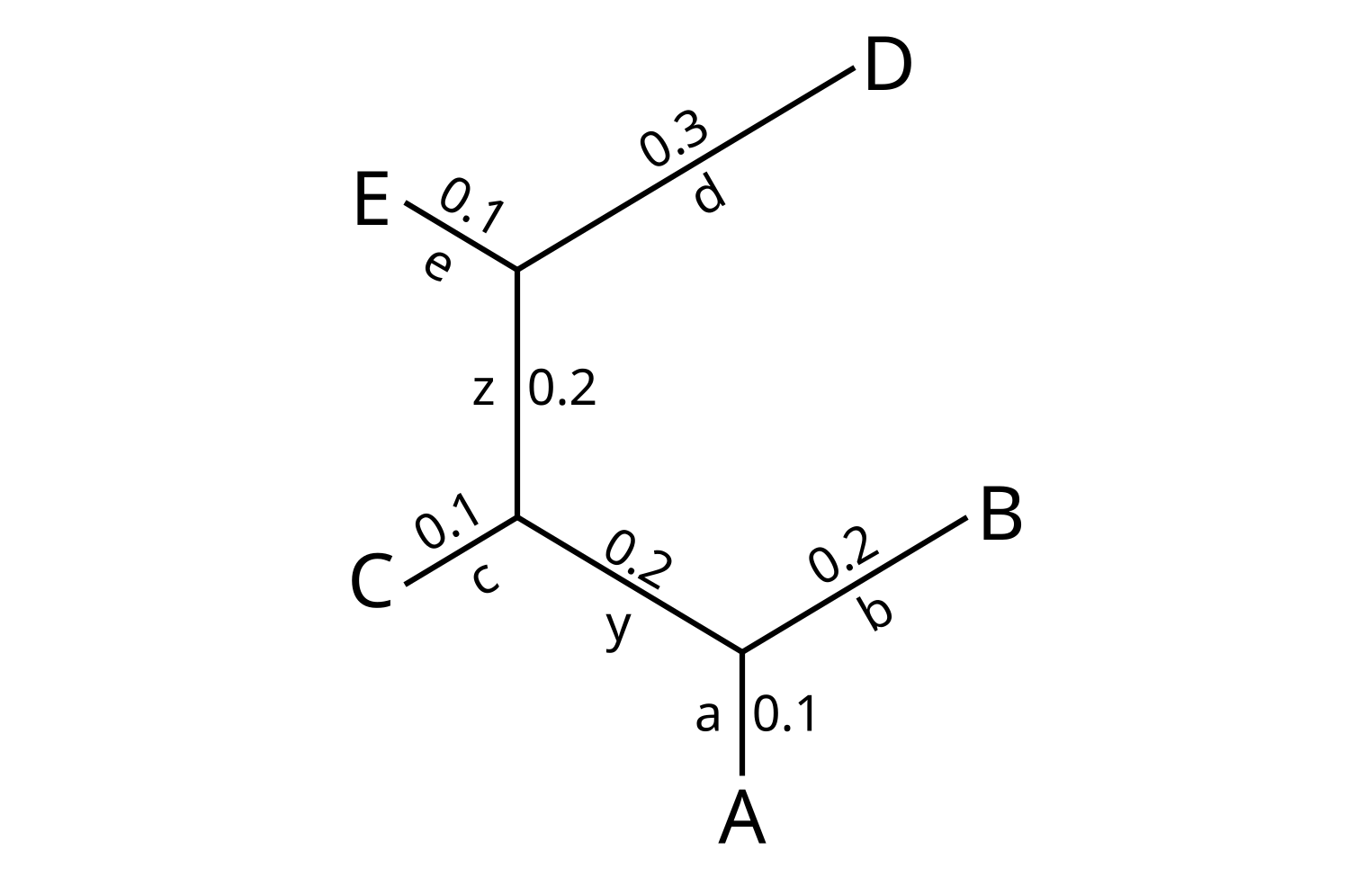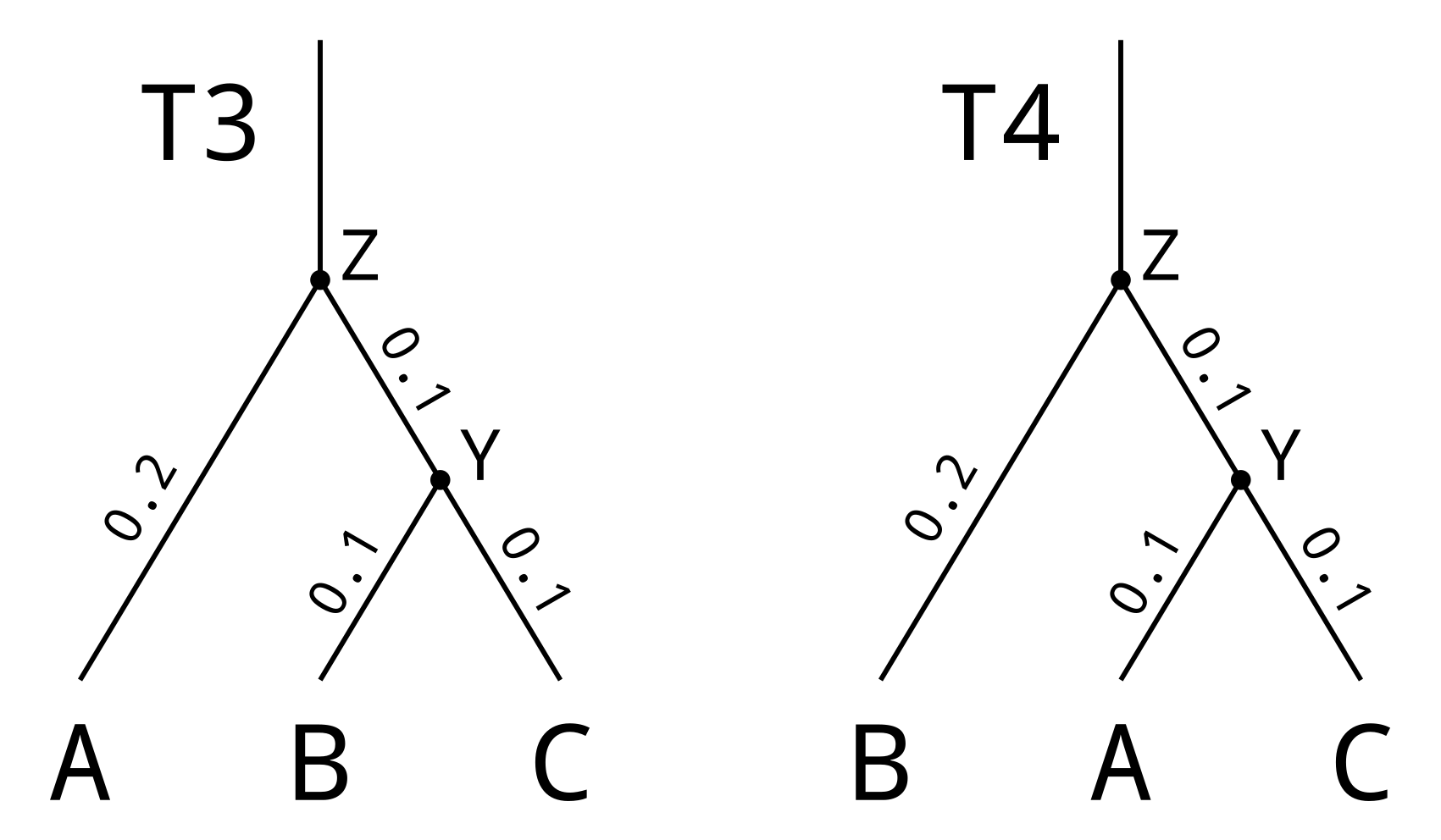# Tree comparisons

The crudest way to compare two trees is to somehow quantify the difference between them as a single number. There are many methods of quantifying the difference, and most of them are metrics which return a distance (according to the mathematical definition). Other functions violate one or more of the conditions for being a metric.

Some of the methods for comparing unrooted trees are:

All the above with the exception of BHV distance also have rooted tree equivalents. For example, rooted NNI (rNNI), rooted SPR (rSPR), rooted branch score (RBS) et cetera. BHV has an ultrametric equivalent, τ-distance. Here I will explain a few of the most commonly used measures.

# Unweighted Robinson-Foulds

Given an unrooted tree, each branch defines a split or bipartition. If we arbitrarily designate one end of the branch (or edge) as the “head”, and the opposite end as the “tail”, we can define the split as every leaf that can be reached through the head node vs every leaf that can be reached through the tail node.

Consider this first example tree, which I will call T1:There are five leaf branches in the tree, which define the splits:

• a = A|BCDE
• b = B|ACDE
• c = C|ABDE
• d = D|ABCE
• e = E|ABCD

There are two internal branches, which define the splits:

• z = ABC|DE

Now consider a second example tree, which I will call T2:The leafsets of the two trees are identical, so the set of splits defined by the leaf branches are identical for the two trees. However, one of the the two internal branches are different:

• y = AB|CDE

The Robinson-Foulds distance is the size of the symmetric difference in splits between T1 and T2. That is, if S is a function to return the splits in a tree, the symmetric difference is |S(T1) - S(T2)| + |S(T2) - S(T1)|. In plain English, this is the number of branches in T1 which define a split absent from T2, plus the number of branches in T2 which define a split absent from T1.

In our example trees, the branch y of T1 (T1y) defines the split BC|ADE which is only present in T1, and the branch y of T2 (T2y) defines the split AB|CDE which is only present in T2. All other branches are present in both trees. Therefore the Robinson-Foulds distance is:

|S(T1) - S(T2)| + |S(T2) - S(T1)| = |{T1y}| + |{T2y}| = 1 + 1 = 2.

# Weighted Robinson-Foulds

For weighted Robinson-Foulds, instead of incrementing the distance by 1 for each branch only seen in one tree, the distance is incremented by the length of the branch. If L is a function to return the length of a branch, the weighted Robinson-Foulds distance between T1 and T2 will be:

L(T1y) + L(T2y) = 0.2 + 0.2 = 0.4

# Rooted Robinson-Foulds

Robinson-Foulds distances can be used for rooted trees by counting clades rather than splits. Consider the following rooted trees:Each node in a rooted tree corresponds to a single rootward branch (which extends upwards from the node when we draw the tree in the above orientation), and defines a “clade” of leaves below the node. The leaf nodes of T3 define the following clades:

• A = {A}
• B = {B}
• C = {C}

That is, each leaf node defines a clade consisting solely of itself. Each internal node in T3 defines the following clades:

• Y = {B, C}
• Z = {A, B, C}

As the leafset of T4 is identical to T3, not only will by definition all the leaf nodes occur in both trees, but the clade defined by the root node T4Z will equal the clade defined by T3Z. However in this example the clade {B, C} defined by T3Y does not occur in T4. And the clade {A, C} defined by T4Y does not occur in T3. The rooted Robinson-Foulds distance will therefore be 2.

The weighted rooted Robinson-Foulds distance is the sum of rootward branch lengths for all nodes which define a clade appearing in only one of the trees. Therefore in the context of rooted trees, the L(x) function will be defined as returning the length of the rootward branch connected to node x. Although the root branch typically (but not always) has an undefined length, as long as the leafsets of both trees are identical, the root nodes of both trees will be identical and not contribute to the total.

The weighted rooted Robinson-Foulds distance for T3 and T4 comes to:

L(T1Y) + L(T2Y) = 0.1 + 0.1 = 0.2.

# Branch score

All forms of Robinson-Foulds distances only penalize differences in tree topology. Another measure, “branch score”, penalizes differences in branch length regardless of whether the local or global topology is identical or not.

The simplest branch score measure sums the absolute differences in branch lengths between two trees. Consider that for the unrooted example, there were 6 branches in common between the two trees. We can calculate the differences in branch lengths:

• L(T1a) - L(T2a) = 0.1 - 0.1 = 0.0
• L(T1b) - L(T2b) = 0.1 - 0.2 = -0.1
• L(T1c) - L(T2c) = 0.2 - 0.1 = 0.1
• L(T1d) - L(T2d) = 0.3 - 0.3 = 0.0
• L(T1e) - L(T2e) = 0.1 - 0.1 = 0.0
• L(T1z) - L(T2z) = 0.2 - 0.2 = 0.0

And two branches only found in one tree:

• L(T1y) = 0.2
• L(T2y) = 0.2

The branch score difference of the two trees is the sum of the absolute differences plus the sum of lengths of branches found in only one tree. This comes to:

|0.0| + |-0.1| + |0.1| + |0.0| + |0.0| + |0.0| + 0.2 + 0.2 = 0.6

# Rooted branch score

The rooted branch score (RBS) is similar to branch score, but instead is composed of the difference in rootward branch lengths, which are for the examples in this post:

• L(T3A) - L(T4A) = 0.2 - 0.1 = 0.1
• L(T3B) - L(T4B) = 0.1 - 0.2 = -0.1
• L(T3C) - L(T4C) = 0.1 - 0.1 = 0.0

Plus the lengths of the rootward branch lengths for nodes defining clades only found in one tree:

• L(T3Y) = 0.1
• L(T4Y) = 0.1

Eventually we arrive at:

|0.1| + |-0.1| + |0.0| + 0.1 + 0.1 = 0.4

# Sum of squares branch score

Instead of the absolute difference, raise each difference in branch length to the power of 2 before summation. The unique branch lengths must also be raised to the power of 2. Alternatively, branches which only appear in one tree can by convention be considered zero length in the other tree. Then you are still calculating the distance in length, but length in one of the trees will be 0.

The sum of squares branch score for T1 and T2 is:

0.02 + -0.12 + 0.12 + 0.02 + 0.02 + 0.02 + 0.22 + 0.22 = 0.1

# Euclidean branch score (don’t use this term, I just made it up)

By treating each branch length as a position along an axis unique to that branch, the branch lengths of a tree can be encoded in Euclidean space. It follows that the square root of the sum of square differences in branch lengths is a Euclidean distance between the two trees! This makes the square root sum of square branch score mathematically attractive.

This branch score for T1 and T2 is therefore sqrt(0.1) = 0.316.

Categories:

Updated: